This example shows how to simulate detection and tracking with a scanning monostatic radar. We'll see how to configure a statistical radar model with mechanical and electronic scanning capabilities, how to set up a scenario management tool to handle platform dynamics and timing, and how to inspect the detections and tracks that are generated.

### Introduction

Statistical radar models such as `radarDataGenerator` offer valuable data for early-stage development of radar systems. The system is modeled from the point of view of its performance characteristics, and a minimal set of parameters is used along with established theory to determine how this system will interact with its environment. This includes specification of fundamental system parameters such as operating frequency, bandwidth, and pulse repetition frequency (PRF), along with important metrics like angle and range resolution, false alarm rate, and detection probability. The radar model has dedicated modes for monostatic, bistatic, and passive operation. The primary output of this model is raw detection data or a sequence of track updates. While time-domain signals are not generated, an emitter can specify a numeric ID for the waveform it's using so that approriate signal gain and rejection can be considered. Detections may be output in the radar frame, or in the scenario frame if the receiving radar has knowledge of the location and orientation of the transmitter. Tracks are generated using one of a variety of tracking and track management algorithms. Data rate is handled by a system update rate specification, so that detections are generated at the appropriate rate.

#### Scenario Management

The model works in tandem with a `radarScenario` to generate detection or track data over time in a dynamic environment. This scenario object not only manages the simulation time, but can handle the passing of data structures between objects in the scene as required when a frame of detection or track update is requested. The radar object is mounted on a platform which handles dynamics, such as position, velocity, and orientation in the scenario frame. These scenario platforms also may contain signature information, which describes how the platform appears to various types of sensors. For our purposes, the `rcsSignature` class will be used to endow target platforms with a radar cross section.

#### Scanning

Scanning functionality is specified with the radar itself, and can be done in a mechanical or electronic style. With mechanical scanning, the pointing direction cannot change instantaneously, but is constrained by a maximum rotation rate in azimuth and elevation. With electronic scanning there is no such constraint, and the scan direction resets at the beginning of each loop. The radar is also allowed to perform mechanical and electronic scanning simultaneously. The figure below demonstrates the difference in scan pattern between these two modes. Notice that with the electronic mode the scan angle is always increasing, and with the mechanical mode the scan position always changes to an adjacent position. The scanning happens in azimuth first because that is the primary scan direction, which is the direction with the greatest extent of scan limit.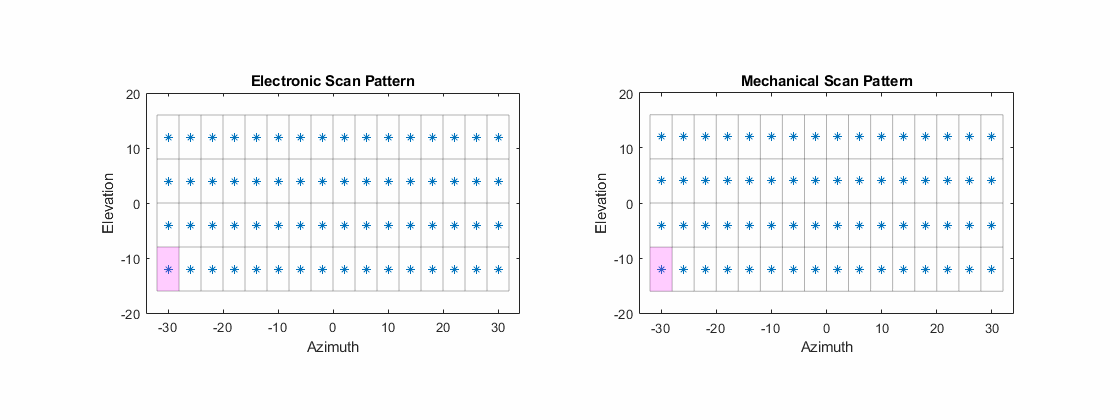### Scenario: Air Surveillance

For this scenario, we'll look at a scanning radar system with its antenna mounted on a tower, as might be used for the tracking of aircraft. We'll scan over a relatively small area, look at a couple inbound platforms with different RCS profiles, and inspect detection and track data. We'll see the effect of SNR and range on our ability to make detections.

Use a 1 GHz center frequency and a bandwidth of 1.5 MHz, to give us a range resolution of about 100 meters. To model a single pulse per scan position, set the update rate equal to the desired PRF. Set the size of the range ambiguity to reflect our PRF choice, and set our upper range limit to twice the size of the range ambiguity to enable detections of objects in the first range ambiguity.

```% Set the seed for repeatability rng(2021); % System parameters c = physconst('lightspeed'); fc = 1e9; % Hz bandwidth = 1.5e6; % Hz prf = 4e3; % Hz updateRate = prf; rangeRes = c/(2*bandwidth); % m rangeAmb = c/(2*prf); % m rangeLims = [0 2*rangeAmb];```

Besides choosing between mechanical and electronic scan modes, the scanning behavior is controlled by the specification of the scan limits and field of view (FoV) in azimuth and elevation. The FoV consists of the azimuth and elevation extent of the observable region at each scan position, similar to a two-sided half-power beamwidth specification. The scan points are chosen so that the total angular extent defined by the scan limit is covered by nonoverlapping segments with size equal to the FoV. Scan limits are specified in the radar's frame, which may be rotated from its platform's frame via the `MountingAngles` property.

We'll scan +/-20 degrees in azimuth and +/-10 degrees in elevation. Set the FoV to 4 degrees azimuth and 8 degrees elevation. Finally, specify the total number of complete scans to simulate, which will dictate the total simulation time.

```% Scanning parameters azLimits = [-20 20]; elLimits = [-10 10]; fov = [4;8]; % deg numFullScans = 20; % number of full scans to simulate```

The total number of scan points can be found as follows. The scan limits denote the minimum and maximum scan angles referenced to the antenna boresight vector, so the total area scanned in one direction is greater than the extent of the scan limits by up to half the FoV in that direction. The pattern is always a rectangular grid over az/el.

```numScanPointsAz = floor(diff(azLimits)/fov(1)) + 1; numScanPointsEl = floor(diff(elLimits)/fov(2)) + 1; numScanPoints = numScanPointsAz*numScanPointsEl;```

A specification of angular resolution separate from FoV will be used, which allows us to model something like monopulse angle estimation. This angular resolution is for determing our ability to discriminate between targets. and is used to derive the actual angle accuracy from the Cramer-Rao lower bound (CRLB) for a monopulse angle estimation with that resolution. Similarly, range resolution determines the minimum spacing in range required to discriminate two targets, but the actual range measurement accuracy comes from the CRLB for range estimation. Exact measureables can be used by turning measurement noise off with the `HasNoise` property. Typically, measurement error does not go to 0 as SNR increases without bound. This can be captured by the "bias" properties, of which there is one for each type of measureable. For our purposes we will leave these at their defaults.

Let our angular resolution be 1/4th the FoV in each direction, which would allow us to discriminate between up to 16 point targets in the FoV at the same range.

`angRes = fov/4;`

#### Reference Target and Radar Loop Gain

Instead of directly specifying things like transmit power, noise power, and specifics of the detection algorithm used, this radar model uses the concept of radar loop gain to translate target RCS and range into an SNR, which is translated directly into a detection probability. Radar loop gain is an important property of any radar system, and is computed with a reference target, which consists of a reference range and RCS, and a receiver operating characteristic (ROC) for given detection and false alarm probabilities.

We'll use the default detection probability of 90% for a reference target at 20 km and 0 dBsm. We'll use the default false alarm rate of 1e-6 false alarms per resolution cell per update.

```refTgtRange = 20e3; refTgtRCS = 0; detProb = 0.9; faRate = 1e-6;```

Let's now construct our radar using the parameters specified above. Use the lower scan limit in elevation to set the pitch mounting angle of the radar from its platform. This points our boresight up so that the lower edge of the scanning area is parallel to the ground (that is, none of our scan points will point the radar towards the ground).

`pitch = elLimits(1); % mount the antenna rotated upwards`

Enable elevation angle measurements, false alarm generation, and range ambiguities with the corresponding `Has...` property. To model a radar that only measures range and angle, set `HasRangeRate` to false. To get detections in scenario coordinates, our radar needs to be aware of the orientation of the transmitter. Because we're using a monostatic radar, this can be achieved by enabling inertial navigation system (INS) functionality with the `HasINS` property.

```% Create radar object sensorIndex = 1; % a unique identifier is required radar = radarDataGenerator(sensorIndex,'UpdateRate',updateRate,... 'DetectionMode','Monostatic','ScanMode','Electronic','TargetReportFormat','Detections','DetectionCoordinates','scenario',... 'HasElevation',true,'HasINS',true,'HasRangeRate',false,'HasRangeAmbiguities',true,'HasFalseAlarms',true,... 'CenterFrequency',fc,'Bandwidth',bandwidth,... 'RangeResolution',rangeRes,'AzimuthResolution',angRes(1),'ElevationResolution',angRes(2),... 'ReferenceRange',refTgtRange,'ReferenceRCS',refTgtRCS,'DetectionProbability',detProb,'FalseAlarmRate',faRate,... 'RangeLimits',rangeLims,'MaxUnambiguousRange',rangeAmb,'ElectronicAzimuthLimits',azLimits,'ElectronicElevationLimits',elLimits,... 'FieldOfView',fov,'MountingAngles',[0 pitch 0]);```

The provided helper class can be used to visualize our scan pattern. We'll also use this plotter to animate the scan pattern in the simulation loop.

```scanplt = helperScanPatternDisplay(radar); scanplt.makeOverviewPlot;```Note that our scan points in azimuth extent exactly to the specified azimuth scan limits, while the elevation scan points are pulled in slightly as needed to avoid overlapping or redundant scan points.

Calculate the total number of range-angle resolution cells for our system, and the total number of frames to be used for detection. We can then compute the expected number of false alarms by multiplying the false alarm rate by the total number of resolution cells interrogated throughout the simulation.

```numResolutionCells = diff(rangeLims)*prod(fov)/(rangeRes*prod(angRes)); numFrames = numFullScans*numScanPoints; % total number of frames for detection expNumFAs = faRate*numFrames*numResolutionCells % expected number of FAs```
```expNumFAs = 7.9200 ```

#### Scenario and Target

We'll use `radarScenario` to manage the top-level simulation flow. This object provides functionality to add new platforms and quickly generate detections from all sensor objects in the scenario. It also manages the flow of time in the simulation. Use the total number of frames calculated above to find the required `StopTime`, and to adaptively update simulation time based on the update rate of objects in the scene, set the scenario update rate to 0.

```% Create scenario stopTime = numFullScans*numScanPoints/prf; scene = radarScenario('StopTime',stopTime,'UpdateRate',0);```

Use the `platform` method to generate new platform objects, add them to the scene, and return a handle for access to the platform properties. Each platform has a unique ID which is assigned by the scenario upon construction. For a static platform, only the Position property needs to be set. To simulate a standard constant-velocity kinematic trajectory, use `kinematicTrajectory` and specify position and velocity.

Let's create two target platforms at different ranges, both inbound at different speeds and angles.

```% Create platforms rdrPlat = platform(scene,'Position',[0 0 12]); % place 12 m above the origin tgtPlat(1) = platform(scene,'Trajectory',kinematicTrajectory('Position',[38e3 6e3 10e3],'Velocity',[-380 -50 0])); tgtPlat(2) = platform(scene,'Trajectory',kinematicTrajectory('Position',[25e3 -3e3 1e3],'Velocity',[-280 10 -10]));```

To enable convenience detection methods and automatic advancement of simulation time, the scenario needs to be aware of the radar object constructed earlier. To do this, mount the radar to the platform by populating the platform's Sensors property. To mount more than one sensor on a platform, the Sensors property may be a cell array of sensor objects.

```% Mount radar to platform rdrPlat.Sensors = radar;```

The `rcsSignature` class can be used to specify platform RCS as a function of aspect angle. For a simple constant-RCS signature, simply set the `Pattern` property to a scalar value. Let one platform be 20 dBsm and the other 4 dBsm to demonstrate the difference in detectability.

```% Set target platform RCS profiles tgtRCS = [20, 4]; % dBsm tgtPlat(1).Signatures = rcsSignature('Pattern',tgtRCS(1)); tgtPlat(2).Signatures = rcsSignature('Pattern',tgtRCS(2));```

#### Detectability

Let's take a look at the theoretical SNR that can be expected for out targets. Because we're using a simple kinematic trajectory, we can compute truth position and range as such:

```time = (0:numFrames-1).'/updateRate; tgtPosTruth(:,:,1) = tgtPlat(1).Position + time*tgtPlat(1).Trajectory.Velocity; tgtPosTruth(:,:,2) = tgtPlat(2).Position + time*tgtPlat(2).Trajectory.Velocity; tgtRangeTruth(:,1) = sqrt(sum((tgtPosTruth(:,:,1)-rdrPlat.Position).^2,2)); tgtRangeTruth(:,2) = sqrt(sum((tgtPosTruth(:,:,2)-rdrPlat.Position).^2,2));```

We can then use the known target RCS and the radar loop gain computed by the radar model to get the truth SNR for each target:

`tgtSnr = tgtRCS - 40*log10(tgtRangeTruth) + radar.RadarLoopGain;`

The minimum SNR required for detection given a magnitude-only detection scheme such as CFAR, given our probability of detection and false alarm rate is

`minSnr = 20*log10(erfcinv(2*radar.FalseAlarmRate) - erfcinv(2*radar.DetectionProbability)); % dB`

Going just a bit further, we can compute our minimum range for detection:

`Rd = 10.^((tgtRCS - minSnr + radar.RadarLoopGain)/40); % meters`

Let's compare our targets' mean range and SNR to the minimum range and SNR thresholds. The total travel distance of our targets is small enough that the range-based SNR does not change significantly.

`snrMargin = mean(tgtSnr,1) - minSnr`
```snrMargin = 1×2 8.0827 0.0019 ```
`rangeMargin = (Rd - mean(tgtRangeTruth,1))/1e3 % km`
```rangeMargin = 1×2 23.5299 0.0028 ```

The first target is about 8 dB brighter than needed for detection with the given CFAR parameters, while the second target is considered barely detectable.

### Simulate Detections

The primary simulation loop starts by calling `advance` on the scenario object. This method steps the scenario forward to the next time at which it's been determined an object in the scene requires an update, and returns false when the specified stop time is reached, exiting the loop. Note that the first call to `advance` does not step the simulation time, so that the first frame of data collection may occur at 0 s. As an alternative to using `advance`, `scene.SimulationStatus` can be checked to determine if the sim has run to completion.

Inside the loop, the detection and track generation methods may be called for any sensor object individually (see the sensor `step` method), but convenience methods exist to generate detections and tracks for all sensors and from all targets in the scene with one function call. Because we only have one sensor, `detect(scene)` is a good solution to get a list of detections for all targets in the scene. By initiating the generation of detections at the top level like this, the scene itself will handle passing required timing, INS, and other configuration data to the sensor. The visualization class used earlier will be used here as well to animate the scan pattern.

```allDets = []; % initialize list of all detections generated lookAngs = zeros(2,numFrames); simTime = zeros(1,numFrames); frame = 0; % current frame while advance(scene) % advance until StopTime is reached % Increment frame index and record simulation time frame = frame + 1; simTime(frame) = scene.SimulationTime; % Generate new detections dets = detect(scene); % Record look angle for inspection lookAngs(:,frame) = radar.LookAngle; % Update coverage plot scanplt.updatePlot(radar.LookAngle); % Compile any new detections allDets = [allDets;dets]; end```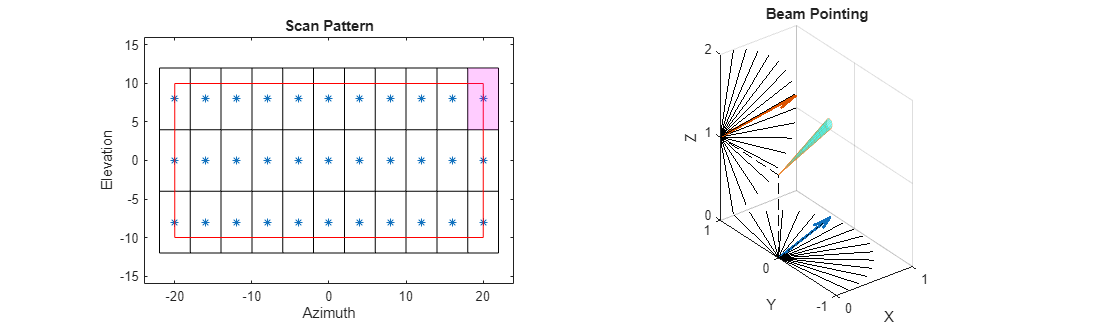#### Scan Behavior

Plot the recorded look angles for the first two complete scans to inspect the pattern. The radar scans first in azimuth, and steps up in elevation at the end of each azimuth scan, instantaneously resetting the look angle at the start of each new scan.

```figure; plot(simTime(1:2*numScanPoints)*1e3,lookAngs(:,1:2*numScanPoints)); xlabel('Time (ms)'); ylabel('Look Angle (deg)'); legend('Azimuth','Elevation'); title('Look Angles');```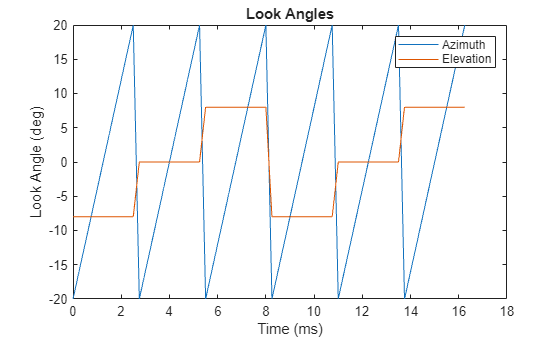#### Detections

Let's inspect the contents of an `objectDetection` as output by our radar.

`allDets{1} % look at the first detection`
```ans = objectDetection with properties: Time: 7.5000e-04 Measurement: [3x1 double] MeasurementNoise: [3x3 double] SensorIndex: 1 ObjectClassID: 0 MeasurementParameters: [1x1 struct] ObjectAttributes: {[1x1 struct]} ```

`Time` is the simulation time at which the detection was generated. `SensorIndex` tells us which sensor this detection was generated by (which is important when we have multiple sensors and are using the scene-level detect method). `Measurement` is the measurable value associated with the detection. The format depends on the choice of our detection mode and output coordinates. `MeasurementNoise` gives the variance or covariance of the measurement and is used by tracker models. Because we're outputting detections in scenario coordinates, the measurement field is simply the estimated position vector of the target, and the measurement noise gives the covariance of that position estimate.

The `ObjectAttributes` field contains some useful metadata:

`allDets{1}.ObjectAttributes{1}`
```ans = struct with fields: TargetIndex: 3 SNR: 12.5894 ```

This tells us that the detection came from the platform with index 2, which is the unique ID assigned by the scenario to our first target platform (the second platform to be added to the scene). We also see the SNR of this detection is about what we expected for our first target. If the detection is a false alarm, TargetIndex will be -1, which is an invalid platform ID. Compile the target index, SNR, and time of each detection.

```detTgtIdx = cellfun(@(t) t.ObjectAttributes{1}.TargetIndex, allDets); detTgtSnr = cellfun(@(t) t.ObjectAttributes{1}.SNR, allDets); detTime = cellfun(@(t) t.Time, allDets); firstTgtDet = find(detTgtIdx == tgtPlat(1).PlatformID); secondTgtDet = find(detTgtIdx == tgtPlat(2).PlatformID);```

We can find the total number of FA detections with:

`numFAs = numel(find(detTgtIdx==-1))`
```numFAs = 8 ```

which is close to the expected number of FAs computed earlier.

Collect the measurement data across detections and plot along with truth positions for the two target platforms.

```tgtPosObs = cell2mat(cellfun(@(t) t.Measurement,allDets,'UniformOutput',0).'); subplot(1,2,1); helperPlotPositionError( tgtPosTruth(:,:,1),time,tgtPosObs(:,firstTgtDet),detTime(firstTgtDet),scene.SimulationTime ); title('Target 1 Position Error'); subplot(1,2,2); helperPlotPositionError( tgtPosTruth(:,:,2),time,tgtPosObs(:,secondTgtDet),detTime(secondTgtDet),scene.SimulationTime ); title('Target 2 Position Error'); set(gcf,'Position',get(gcf,'Position')+[0 0 600 0]);```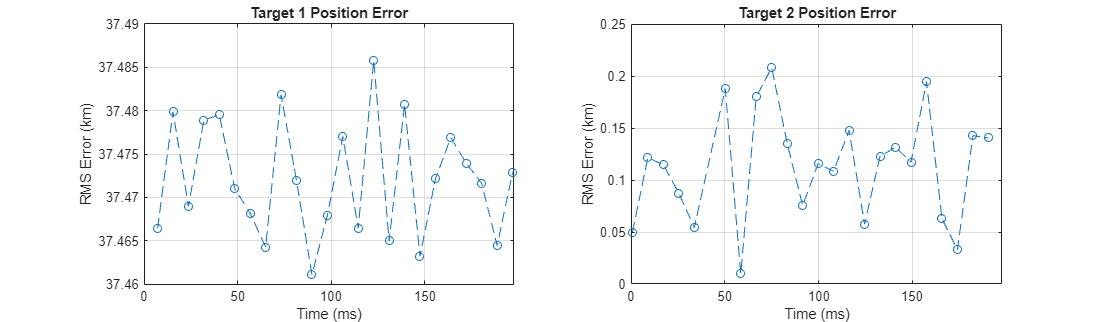Most of our detections originate from the first target, which is unsurprising considering the detectability analysis earlier. However, it was not detected on every frame, and the second target has generated many detections despite having a low SNR. Though the first target is easily detected, the measurement error is large because the target is in our second range ambiguity and no disambiguation has been performed, whereas our second target, when detected, shows position error that is consistent with our 100 meter range resolution and relatively poor angle resolution.

Let's also look at the total variance of the detections against SNR. The total variance is the sum of the marginal variances in each cartesian direction (X, Y, and Z). This variance includes the effects of estimation in range-angle space along with the transformation of those statistics to scenario coordinates.

```detTotVar = cellfun(@(t) trace(t.MeasurementNoise),allDets); figure; subplot(1,2,1); helperPlotPositionTotVar( detTgtSnr(firstTgtDet),detTotVar(firstTgtDet) ); title('First Target'); subplot(1,2,2); helperPlotPositionTotVar( detTgtSnr(secondTgtDet),detTotVar(secondTgtDet) ); title('Second Target'); set(gcf,'Position',get(gcf,'Position')+[0 0 600 0]);```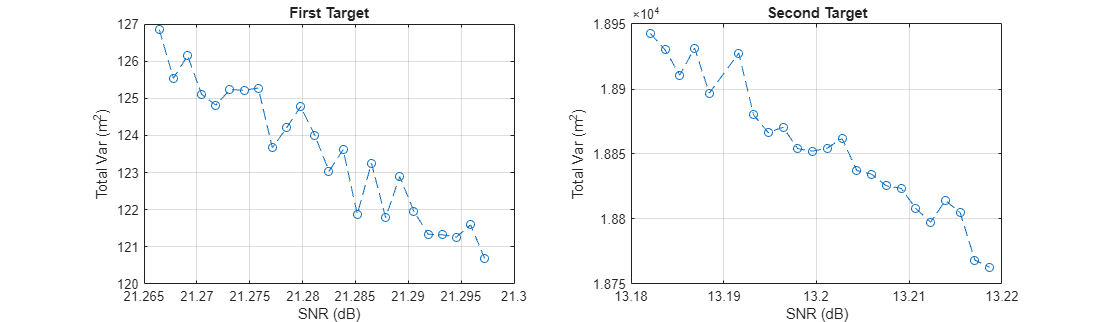Note that although the absolute position error for the first target was greater due to the range ambiguity, the lower measurement variance reflects both the greater SNR of the first target and its apparently short range.

### Simulate Tracks

The `radarDataGenerator` is also capable of performing open-loop tracking. Instead of outputting detection objects, it can output track updates.

Let's run the simulation again, but this time we'll let our radar model output tracks directly. Firstly, re-set some properties of the radar model to generate tracks in the scenario frame.

```release(radar); % unlock for re-simulation radar.TargetReportFormat = 'Tracks'; radar.TrackCoordinates = 'Scenario';```

Tracking can be performed by a number of different algorithms. There are simple alpha-beta filters and Kalman filters of the linear, extended, and unscented variety, along with constant-velocity, constant-acceleration, and constant-turnrate motion models. The algorithm to be used is specified with the `FilterInitializationFcn` property. This is a function handle or the name of a function in a character vector, i.e. `@initcvekf` or `'initcvekf',` that takes an initial detection as input and returns an initialized tracker object. Any user-defined function with the same signature may be used. We'll use the supplied constant-velocity extended Kalman filter.

`radar.FilterInitializationFcn = @initcvekf;`

The overall structure of the sim loop is the same, however we must call the radar's `step` function manually. The required inputs are a target poses struct, acquired by calling `targetPoses` on our radar platform, the INS struct, acquired by calling `pose` on the radar platform, and the current simulation time.

```restart(scene); % restart scenario allTracks = []; % initialize list of all track data while advance(scene) % Generate new track data tgtPoses = targetPoses(rdrPlat); ins = pose(rdrPlat); tracks = radar(tgtPoses,ins,scene.SimulationTime); % Compile any new track data allTracks = [allTracks;tracks]; end```

Inspecting a track object:

`allTracks(1) % look at first track update`
```ans = objectTrack with properties: TrackID: 1 BranchID: 0 SourceIndex: 1 UpdateTime: 0.0093 Age: 34 State: [6x1 double] StateCovariance: [6x6 double] StateParameters: [1x1 struct] ObjectClassID: 0 TrackLogic: 'History' TrackLogicState: [1 1 0 0 0] IsConfirmed: 1 IsCoasted: 0 IsSelfReported: 1 ObjectAttributes: [1x1 struct] ```

`TrackID` is the unique identifier of the track file, and `SourceIndex` is the ID of the tracker object reporting the track, which is useful when you have multiple trackers in the scene. When a multi-hypothesis tracker is used, `BranchID` gives the index of the hypothesis used. The `State` field contains the state information on this update. Because we're using a constant-velocity model and scenario-frame track coordinates, `State` consists of the position and velocity vectors. `UpdateTime` gives the time at which this track update was generated. Another important property, `IsCoasted`, tells you if this track update used a fresh target detection to update the filter, or if it's simply propagated forward in time from the last target detection (the track is "coasted").

The ObjectAttributes structure gives the ID of the platform whose signature is being detected, and the SNR of that signature during the update.

`allTracks(1).ObjectAttributes`
```ans = struct with fields: TargetIndex: 3 SNR: 18.5910 ```

Collect the target index and update time. Also check how many detections were used to update our tracks.

```trackTgtIdx = arrayfun(@(t) t.TargetIndex,[allTracks.ObjectAttributes]); updateTime = [allTracks.UpdateTime]; firstTgtTrack = find(trackTgtIdx == tgtPlat(1).PlatformID); secondTgtTrack = find(trackTgtIdx == tgtPlat(2).PlatformID); numUpdates = numel(find(~[allTracks.IsCoasted])) % total number of track updates with new detection data```
```numUpdates = 31 ```

This is roughly the number of non-FA detections generated in the first part.

Let's look at the reported positions in the XY plane and their covariances, along with truth position data. Because the first target is in the first range ambiguity and no disambiguation is performed, let's first find the "truth" ambiguous position for the first target as such:

```los = tgtPosTruth(:,:,1) - rdrPlat.Position; R = sqrt(sum(los.^2,2)); los = los./R; Ramb = mod(R,rangeAmb); tgtPosTruthAmb = rdrPlat.Position + los.*Ramb;```

Now use the provided helper function to plot a visualization of the position track.

```figure; subplot(1,2,1); helperPlotPositionTrack( tgtPosTruthAmb,allTracks(firstTgtTrack) ); title('First Target Track'); subplot(1,2,2); helperPlotPositionTrack( tgtPosTruth(:,:,2),allTracks(secondTgtTrack) ); title('Second Target Track'); set(gcf,'Position',get(gcf,'Position')+[0 0 600 0]);```In both cases, the position estimates start by converging on the truth (or range-ambiguous truth) trajectory. For the first target we have more track updates and the final state appears closer to steady-state convergence. Looking at the covariance contours, we see the expected initial decrease in size as the tracking filter warms up.

To take a closer look at our tracking performance, plot the magnitude of the position estimation error for both targets, and indicate which samples were updated with target detections.

```figure; subplot(1,2,1); helperPlotPositionTrackError( tgtPosTruthAmb,time,allTracks(firstTgtTrack),updateTime(firstTgtTrack) ); title('First Target Ambiguous Position Error'); subplot(1,2,2); helperPlotPositionTrackError( tgtPosTruth(:,:,2),time,allTracks(secondTgtTrack),updateTime(secondTgtTrack) ); title('Second Target Position Error'); set(gcf,'Position',get(gcf,'Position')+[0 0 600 0]);```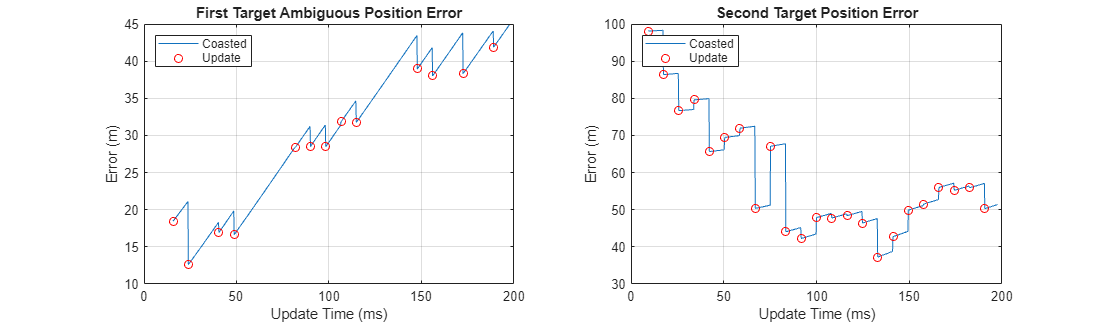For the first target, position error decreases on samples that had a new detection to update the track file (indicated by a red circle). Since the second target had much lower SNR, the error in some individual detections was great enough to increase the position error of the track. Despite this, the second target track position error has a small downward trend, indicating that the estimated state will likely converge. However, we see an issue with our ability to track the first target. Because it is in the first range ambiguity and has a component of motion perpendicular to the line of sight, the apparent velocity is actually changing, and the constant-velocity Kalman filter is unable to converge.

### Conclusion

In this example we configured a statistical radar system for use in the simulation and analysis of target detectability and trackability. We saw how to use the scan limits and field of view properties to define a scan pattern, how to run the radar model with a scenario management tool, and how to inspect the generated detections and tracks.

### Helper Functions

```function helperPlotPositionError( tgtPosTruth,time,tgtPosObs,detTime,T ) tgtPosTruthX = interp1(time,tgtPosTruth(:,1),detTime).'; tgtPosTruthY = interp1(time,tgtPosTruth(:,2),detTime).'; tgtPosTruthZ = interp1(time,tgtPosTruth(:,3),detTime).'; err = sqrt((tgtPosTruthX - tgtPosObs(1,:)).^2+(tgtPosTruthY - tgtPosObs(2,:)).^2+(tgtPosTruthZ - tgtPosObs(3,:)).^2); plot(detTime*1e3,err/1e3,'--o'); grid on; xlim([0 T*1e3]); ylabel('RMS Error (km)'); xlabel('Time (ms)'); end function helperPlotPositionTotVar( detTgtSnr,detTotVar ) plot(detTgtSnr,detTotVar,'--o'); grid on; xlabel('SNR (dB)'); ylabel('Total Var (m^2)'); end function helperPlotPositionTrack( tgtPosTruth,tracks ) plot(tgtPosTruth(:,1)/1e3,tgtPosTruth(:,2)/1e3); hold on; updateIdx = find(~[tracks.IsCoasted]); theta = 0:0.01:2*pi; for ind = 1:2:numel(updateIdx) % plot update T = tracks(updateIdx(ind)); plot(T.State(1)/1e3,T.State(3)/1e3,'*black'); sigma = T.StateCovariance([1 3],[1 3]); [evec,eval] = eigs(sigma); mag = sqrt(diag(eval)).'; u = evec(:,1)*mag(1); v = evec(:,2)*mag(2); x = cos(theta)*u(1) + sin(theta)*v(1); y = cos(theta)*u(2) + sin(theta)*v(2); plot(x/1e3 + T.State(1)/1e3,y/1e3 + T.State(3)/1e3,'magenta'); end hold off; grid on; xlabel('X (km)'); ylabel('Y (km)'); legend('Truth Position','Position Estimates','Covariance','Location','northwest'); end function helperPlotPositionTrackError( tgtPosTruth,time,tracks,updateTime ) state = [tracks.State]; sx = state(1,:); sy = state(3,:); sz = state(5,:); x = interp1(time,tgtPosTruth(:,1),updateTime); y = interp1(time,tgtPosTruth(:,2),updateTime); z = interp1(time,tgtPosTruth(:,3),updateTime); err = sqrt((x-sx).^2+(y-sy).^2+(z-sz).^2); isUpdate = ~[tracks.IsCoasted]; plot(updateTime*1e3,err); hold on; plot(updateTime(isUpdate)*1e3,err(isUpdate),'or'); hold off; grid on; xlabel('Update Time (ms)'); ylabel('Error (m)') legend('Coasted','Update','Location','northwest'); end```